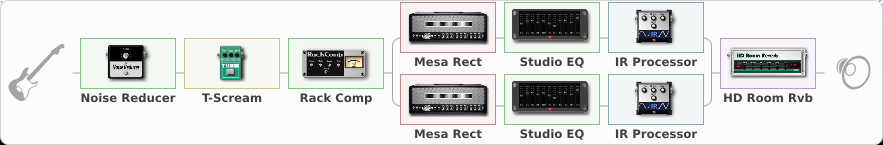# Black Metal Mega Stack

Discussion in 'ToneLib-GFX presets' started by Tetragramm1983, Jul 12, 2020.

1. ### Tetragramm1983Active Member

Black Metal Mega Stack

Preset name: Black Metal Mega Stack

Krank V30+Mesa Oversized 4x12 SM57

Effects chain:Effect: "Noise Reducer" (Dynamics / Filter), active - "yes"
{
"Sens" = 70
"Mode" = Hard
}

Effect: "T-Scream" (Overdrive / Distortion), active - "yes"
{
"Drive" = 5
"Tone" = 41
"Level" = 85
}

Effect: "Rack Comp" (Dynamics / Filter), active - "yes"
{
"Threshold (dB)" = -13
"Ratio" = 4
"Attack" = Fast
"Release (ms)" = 118
"Knee" = 6
"Level (dB)" = 8
}

Effect: "Splitter" (Dynamics / Filter), active - "yes"
{
"A-Bypass" = Off
"A-Pan" = 0
"A-Level" = 55
"B-Bypass" = Off
"B-Pan" = 0
"B-Level" = 55

'A' branch:
{

Effect: "Mesa Rect" (Amp simulators), active - "yes"
{
"Gain" = 70
"Bass" = 35
"Middle" = 90
"Treble" = 81
"Presence" = 85
"Master" = 60
"Level (dB)" = 6
}

Effect: "Studio EQ" (Dynamics / Filter), active - "yes"
{
"31 Hz" = -15
"62 Hz" = -15
"125 Hz" = -4
"250 Hz" = 2
"500 Hz" = 4
"1 kHz" = 4
"2 kHz" = 4
"4 kHz" = -6
"8 kHz" = 4
"16 kHz" = 3
"Level (dB)" = 2
}

Effect: "IR Processor" (Cabinets), active - "yes"
{
"IR" = Mesa Oversized 4x12 V30 SM57 01
"Low Cut (Hz)" = 100
"Hi Cut (kHz)" = 17.0
"Mix" = 100
"Level (dB)" = 2
}
}
'B' branch:
{

Effect: "Mesa Rect" (Amp simulators), active - "yes"
{
"Gain" = 55
"Bass" = 25
"Middle" = 50
"Treble" = 85
"Presence" = 85
"Master" = 60
"Level (dB)" = 3
}

Effect: "Studio EQ" (Dynamics / Filter), active - "yes"
{
"31 Hz" = -15
"62 Hz" = -15
"125 Hz" = -2
"250 Hz" = 4
"500 Hz" = -8
"1 kHz" = -4
"2 kHz" = 6
"4 kHz" = -4
"8 kHz" = 6
"16 kHz" = 6
"Level (dB)" = 2
}

Effect: "IR Processor" (Cabinets), active - "yes"
{
"IR" = To Krank V30 cab
"Low Cut (Hz)" = 99
"Hi Cut (kHz)" = 16.5
"Mix" = 100
"Level (dB)" = 2
}
}
}

Effect: "HD Room Rvb" (Reverberation), active - "yes"
{
"Time" = 6.6
"PreLPF" = 80
"PreDelay" = 65
"HiDamp" = 68
"LoGain" = -3.5
"Mix" = 41
}

Note: You will need to download and install the ToneLib-GFX software to use the preset.

#### Attached Files:

• ###### Black_Metal_Mega_Stack.tlgfx
File size:
16.4 KB
Views:
4,033
ananasnv, FoetopsY, loshara and 15 others like this.
2. ### Tetragramm1983Active Member

Cabs in Description! Like me or leave me a message!
My Black Metal Projects:
satandestroyer.bandcamp.com

#### Attached Files:

File size:
2.4 KB
Views:
58
• ###### Mesa Oversized 4x12 V30 SM57 01.wav
File size:
64.7 KB
Views:
52
FoetopsY likes this.